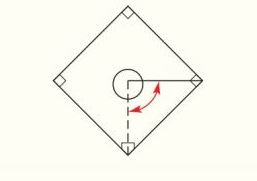Chapter 2.CR, Problem 47CRElementary Geometry For College St...

7th Edition
Alexander + 2 others
ISBN: 9781337614085

Solutions

Chapter
SectionElementary Geometry For College St...

7th Edition
Alexander + 2 others
ISBN: 9781337614085
Textbook Problem

Through what approximate angle of rotation must a baseball pitcher turn when throwing to first base rather than home plate?To determine

To find:

The approximate angle of rotation must a baseball pitcher turn when throwing to first base rather than home plate.

Explanation

Given:

The given figure is,

Approach:

Angle of a circular ball is 360°.

Calculation:

The figure is given by,

Here, we are trying to approximate the measure of angle 1, when the angle of the baseball pitcher repeat four times the turn when throwing to first base ball rather than the home plate, then he reaches his original direction.

From the angle addition postulate,

360°=m1+m2+m3+m4</

Still sussing out bartleby?

Check out a sample textbook solution.

See a sample solution

The Solution to Your Study Problems

Bartleby provides explanations to thousands of textbook problems written by our experts, many with advanced degrees!

Get Started

Given I=EZ,E=240, and Z=15, find I.

Elementary Technical Mathematics

Evaluate the integral: 04x2x2ydy

Calculus: Early Transcendental Functions

Use transformations to sketch the graph of the function. 15. f(x) = cos 2x

Single Variable Calculus: Early Transcendentals, Volume I

5. Find the derivative of each of the following functions. (a) (b)

Mathematical Applications for the Management, Life, and Social Sciences

Evaluate the expression sin Exercises 116. 22212424

Finite Mathematics and Applied Calculus (MindTap Course List)

In Exercises 107-120, factor each expression completely. 112. x2 2x 15

Applied Calculus for the Managerial, Life, and Social Sciences: A Brief Approach

True or False: limx6x3+8x29x+75x34x+30=limx6x35x3.

Study Guide for Stewart's Single Variable Calculus: Early Transcendentals, 8th